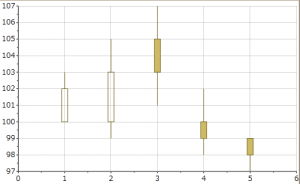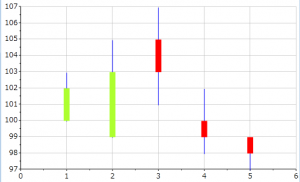You have often seen Financial/Stock Charts which are popularly known as Candle Chart in Technical terms. Candle chart is a line chart where the data point has low/high values. C1Chart for WPF also provide Candle version for its Chart control. This is how a Candle Chart looks like.In this blog we look to customize the Candle chart to fill the BackColor of the individual Candle elements. Now this is easy when it comes to change the color for Bar or Column Charts. You can use the following code to do so.

``````
dataSeries.PlotElementLoaded += (s, e) =>
{
PlotElement pe = (PlotElement)s;
if (pe.DataPoint.PointIndex >= 0)
pe.Fill = new SolidColorBrush(Colors.Blue);
};

``````

However, this does not work for Candle Charts. Above code will fill the Negative Candle element, but it fails for Positive Candles. This is a design standard followed mostly by all type of Chart components. Lets have a quick understanding of the Positive and Negative Candles. Candle sticks usually compose of a body, and an upper and a lower shadow (wick). The area between the open and the close values is called the real body. The wick illustrates the highest and lowest values. The body illustrates the opening and closing trades. If the Close value is higher than it Open value(Positive), body is white or unfilled, with the opening price at the bottom of the body and the closing price at the top. If the Closed values are lower than their Open values(Negative), the body is filled, with the opening price at the top and the closing price at the bottom. Solution to customize the Candle is to replace the original PlotElement with another element like a Rectangle or Border object. Following code snippet shows how you can do so using a Rectangle

``````
private void HighLowOpenCloseSeries_PlotElementLoaded(object sender, EventArgs e)
{
PlotElement pe = sender as PlotElement;

// Retrieve the values of the Candle Element
double open = (double)pe.DataPoint["OpenValues"];
double close = (double)pe.DataPoint["CloseValues"];
double low = (double)pe.DataPoint["LowValues"];
double high = (double)pe.DataPoint["HighValues"];

// Declare a new Rect element to replace the original PlotElement
Rectangle rect = new Rectangle();
// Get the region of the Current PlotElement
Rect rct = (((System.Windows.Shapes.Shape)(pe)).RenderedGeometry).Bounds;

// Get the Parent Container of the PlotElements
Canvas cvs = pe.Parent as Canvas;
// Add the new Rectangle to the Parent Container

// Retrieve the Chart Coordinates based on the High/Low and Open/Close values
// These points are used to position the new Rectangle
Point openPoint = c1Chart1.View.AxisX.PointFromData(new Point(pe.DataPoint.PointIndex + 1, open));
Point closePoint = c1Chart1.View.AxisX.PointFromData(new Point(pe.DataPoint.PointIndex + 1, close));

Point lowPoint = c1Chart1.View.AxisX.PointFromData(new Point(pe.DataPoint.PointIndex + 1, low));
Point HighPoint = c1Chart1.View.AxisX.PointFromData(new Point(pe.DataPoint.PointIndex + 1, high));

Canvas.SetLeft(rect, closePoint.X - (rct.Width / 2));
rect.Width = rct.Width;
rect.Height = lowPoint.Y - closePoint.Y;

// For Positive Candle Values
if (close > open)
{
Canvas.SetTop(rect, closePoint.Y - 1);
rect.Fill = Brushes.GreenYellow;
}
// For Negative Candle Values
else
{
Canvas.SetTop(rect, openPoint.Y - 1);
rect.Fill = Brushes.Red;
}

// Add Line for marking Low/High Points (Candle Wick)
Line ln = new Line();
ln.StrokeThickness = 1;
ln.Stroke = new SolidColorBrush(Colors.Blue);
ln.X1 = lowPoint.X; ln.Y1 = lowPoint.Y;
ln.X2 = HighPoint.X; ln.Y2 = HighPoint.Y;
Above code snippet customizes the Candle elements as shown in the image below.Refer to the attached samples for complete implementation. Download Sample C# Download Sample VB.Net